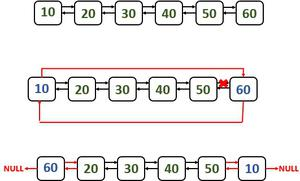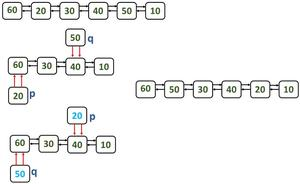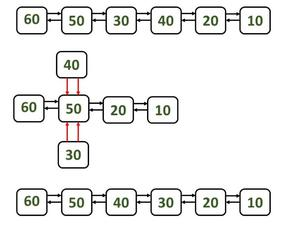# Swap Kth node from beginning with Kth node from end in a Doubly Linked List

• Difficulty Level : Hard
• Last Updated : 15 Apr, 2021

Given a doubly-linked list, the task is to swap Kth node from the beginning with Kth node from the ending.
Note: Please note here the nodes are swapped and not the data in the nodes.
Examples:

Input: DLL = 1 <-> 2 <-> 3 <-> 4 <-> 5 <-> 6, K = 3
Output: 1 2 4 3 5 6
Explanation:
Third node from the beginning(3) is swapped with third node from the ending(4).
Input: DLL = 1 <-> 2 <-> 3 <-> 4 <-> 5, K = 1
Output: 5 2 3 4 1

Approach: The idea is to traverse to the Kth element from the beginning and Kth node from the ending and change the previous and next pointers. Let K1 be the Kth node from beginning and K2 be Kth node from ending. Then:

• The previous node to K2 has to be changed to the previous node of K1.
• The next node to K2 has to be changed to the next node of K1.
• The previous node to K1 has to be changed to the previous node of K2.
• The next node to K1 has to be changed to the next node of K2.

Below is the implementation of the above approach:

## Java

 `// Java implementation of the approach` `public` `class` `GFG {` `    ``// Doubly Linked List implementation``    ``private` `class` `Node {``        ``private` `int` `data;``        ``private` `Node next;``        ``private` `Node previous;` `        ``public` `Node(``int` `data, Node next,``                    ``Node previous)``        ``{``            ``this``.data = data;``            ``this``.next = next;``            ``this``.previous = previous;``        ``}` `        ``public` `int` `getData()``        ``{``            ``return` `data;``        ``}` `        ``public` `void` `setData(``int` `data)``        ``{``            ``this``.data = data;``        ``}` `        ``public` `Node getNext()``        ``{``            ``return` `next;``        ``}` `        ``public` `void` `setNext(Node next)``        ``{``            ``this``.next = next;``        ``}` `        ``public` `Node getPrevious()``        ``{``            ``return` `previous;``        ``}` `        ``public` `void` `setPrevious(Node previous)``        ``{``            ``this``.previous = previous;``        ``}``    ``}` `    ``private` `Node head;``    ``private` `Node tail;` `    ``public` `GFG()``    ``{``        ``this``.head = ``null``;``        ``this``.tail = ``null``;``    ``}` `    ``public` `Node getHead()``    ``{``        ``return` `head;``    ``}` `    ``public` `void` `setHead(Node head)``    ``{``        ``this``.head = head;``    ``}` `    ``public` `Node getTail()``    ``{``        ``return` `tail;``    ``}` `    ``public` `void` `setTail(Node tail)``    ``{``        ``this``.tail = tail;``    ``}` `    ``// Function to replace Kth node from``    ``// beginning with Kth node from end``    ``public` `void` `swapNode(Node headReference,``                         ``Node tailReference, ``int` `k)``    ``{` `        ``// If K is 1, then the first node``        ``// has to be swapped with the``        ``// last node in the doubly linked list``        ``if` `(k == ``1``) {``            ``swapFirstAndLast(headReference,``                             ``tailReference);``            ``return``;``        ``}` `        ``// If k is N, then the last node``        ``// has to be swapped with the``        ``// first node in the doubly linked list``        ``int` `nodeCount = getCount(headReference);``        ``if` `(k == nodeCount) {``            ``swapFirstAndLast(headReference,``                             ``tailReference);``            ``return``;``        ``}` `        ``// If the Kth node from``        ``// the beginning and Kth node``        ``// from the ending are same``        ``if` `(``2` `* k - ``1` `== nodeCount) {``            ``return``;``        ``}` `        ``// fNode represents Kth node``        ``// from the beginning``        ``Node fNode = headReference;``        ``for` `(``int` `i = ``1``; i < k; i++) {``            ``fNode = fNode.getNext();``        ``}``        ``Node fNodePrevious = fNode.getPrevious();``        ``Node fNodeNext = fNode.getNext();` `        ``// sNode represents Kth node``        ``// from the ending``        ``Node sNode = tailReference;``        ``for` `(``int` `i = ``1``; i < k; i++) {``            ``sNode = sNode.getPrevious();``        ``}` `        ``Node sNodePrevious = sNode.getPrevious();``        ``Node sNodeNext = sNode.getNext();` `        ``// Checking if any of the pointers is null``        ``// and interchanging the pointers``        ``if` `(fNodePrevious != ``null` `&& sNode != ``null``) {` `            ``fNodePrevious.setNext(sNode);``            ``sNode.setPrevious(fNodePrevious);``            ``sNode.setNext(fNodeNext);``            ``fNodeNext.setPrevious(sNode);``        ``}``        ``if` `(sNodePrevious != ``null` `&& sNodeNext != ``null``) {` `            ``sNodeNext.setPrevious(fNode);``            ``fNode.setNext(sNodeNext);``            ``sNodePrevious.setNext(fNode);``            ``fNode.setPrevious(sNodePrevious);``        ``}``    ``}` `    ``// Function to swap the first and``    ``// last node in the doubly linked list``    ``private` `void` `swapFirstAndLast(``        ``Node headReference,``        ``Node tailReference)``    ``{``        ``Node headRef = headReference;``        ``Node tailRef = tailReference;` `        ``headReference``            ``= headReference.getNext();``        ``tailReference``            ``= tailReference.getPrevious();` `        ``tailReference.setNext(headRef);``        ``headRef.setPrevious(tailReference);``        ``headRef.setNext(``null``);``        ``this``.setTail(tailReference.getNext());` `        ``headReference.setPrevious(tailRef);``        ``tailRef.setNext(headReference);``        ``tailRef.setPrevious(``null``);``        ``this``.setHead(headReference``                         ``.getPrevious());``    ``}` `    ``// Function to return the number of nodes``    ``// in the linked list``    ``private` `int` `getCount(Node headReference)``    ``{``        ``int` `nodeCount = ``0``;``        ``while` `(headReference != ``null``) {``            ``nodeCount++;``            ``headReference = headReference``                                ``.getNext();``        ``}``        ``return` `nodeCount;``    ``}` `    ``// Function to print the Linked List``    ``public` `void` `printList(Node headReference)``    ``{``        ``if` `(headReference == ``null``) {``            ``System.out.println(``                ``"Doubly linked list is empty"``);``            ``return``;``        ``}``        ``else` `{``            ``while` `(headReference != ``null``) {``                ``System.out.print(``                    ``headReference.getData()``                    ``+ ``" "``);``                ``headReference``                    ``= headReference.getNext();``            ``}``        ``}``    ``}` `    ``// Function to insert a node at``    ``// the end of the doubly linked list``    ``public` `void` `push(``int` `data)``    ``{``        ``Node newNode``            ``= ``new` `Node(data, ``null``, ``null``);` `        ``if` `(head == ``null``) {``            ``head = tail = newNode;``        ``}``        ``else` `{``            ``tail.setNext(newNode);``            ``newNode.setPrevious(tail);``            ``tail = newNode;``        ``}``    ``}` `    ``// Driver code``    ``public` `static` `void` `main(String[] args)``    ``{` `        ``// Creating an object for the class``        ``GFG list = ``new` `GFG();` `        ``// Adding data to the linked list``        ``list.push(``1``);``        ``list.push(``2``);``        ``list.push(``3``);``        ``list.push(``4``);``        ``list.push(``5``);` `        ``// Calling the function``        ``int` `K = ``2``;``        ``list.swapNode(list.getHead(),``                      ``list.getTail(), K);``        ``list.printList(list.getHead());``    ``}``}`

## C#

 `// C# implementation of the approach``using` `System;``public` `class` `GFG {`` ` `    ``// Doubly Linked List implementation``    ``private` `class` `Node {``        ``private` `int` `data;``        ``private` `Node next;``        ``private` `Node previous;`` ` `        ``public` `Node(``int` `data, Node next,``                    ``Node previous)``        ``{``            ``this``.data = data;``            ``this``.next = next;``            ``this``.previous = previous;``        ``}`` ` `        ``public` `int` `getData()``        ``{``            ``return` `data;``        ``}`` ` `        ``public` `void` `setData(``int` `data)``        ``{``            ``this``.data = data;``        ``}`` ` `        ``public` `Node getNext()``        ``{``            ``return` `next;``        ``}`` ` `        ``public` `void` `setNext(Node next)``        ``{``            ``this``.next = next;``        ``}`` ` `        ``public` `Node getPrevious()``        ``{``            ``return` `previous;``        ``}`` ` `        ``public` `void` `setPrevious(Node previous)``        ``{``            ``this``.previous = previous;``        ``}``    ``}`` ` `    ``private` `Node head;``    ``private` `Node tail;`` ` `    ``public` `GFG()``    ``{``        ``this``.head = ``null``;``        ``this``.tail = ``null``;``    ``}`` ` `    ``Node getHead()``    ``{``        ``return` `head;``    ``}`` ` `    ``void` `setHead(Node head)``    ``{``        ``this``.head = head;``    ``}`` ` `    ``Node getTail()``    ``{``        ``return` `tail;``    ``}`` ` `    ``void` `setTail(Node tail)``    ``{``        ``this``.tail = tail;``    ``}`` ` `    ``// Function to replace Kth node from``    ``// beginning with Kth node from end``    ``void` `swapNode(Node headReference,``                         ``Node tailReference, ``int` `k)``    ``{`` ` `        ``// If K is 1, then the first node``        ``// has to be swapped with the``        ``// last node in the doubly linked list``        ``if` `(k == 1) {``            ``swapFirstAndLast(headReference,``                             ``tailReference);``            ``return``;``        ``}`` ` `        ``// If k is N, then the last node``        ``// has to be swapped with the``        ``// first node in the doubly linked list``        ``int` `nodeCount = getCount(headReference);``        ``if` `(k == nodeCount) {``            ``swapFirstAndLast(headReference,``                             ``tailReference);``            ``return``;``        ``}`` ` `        ``// If the Kth node from``        ``// the beginning and Kth node``        ``// from the ending are same``        ``if` `(2 * k - 1 == nodeCount) {``            ``return``;``        ``}`` ` `        ``// fNode represents Kth node``        ``// from the beginning``        ``Node fNode = headReference;``        ``for` `(``int` `i = 1; i < k; i++) {``            ``fNode = fNode.getNext();``        ``}``        ``Node fNodePrevious = fNode.getPrevious();``        ``Node fNodeNext = fNode.getNext();`` ` `        ``// sNode represents Kth node``        ``// from the ending``        ``Node sNode = tailReference;``        ``for` `(``int` `i = 1; i < k; i++) {``            ``sNode = sNode.getPrevious();``        ``}`` ` `        ``Node sNodePrevious = sNode.getPrevious();``        ``Node sNodeNext = sNode.getNext();`` ` `        ``// Checking if any of the pointers is null``        ``// and interchanging the pointers``        ``if` `(fNodePrevious != ``null` `&& sNode != ``null``) {`` ` `            ``fNodePrevious.setNext(sNode);``            ``sNode.setPrevious(fNodePrevious);``            ``sNode.setNext(fNodeNext);``            ``fNodeNext.setPrevious(sNode);``        ``}``        ``if` `(sNodePrevious != ``null` `&& sNodeNext != ``null``) {`` ` `            ``sNodeNext.setPrevious(fNode);``            ``fNode.setNext(sNodeNext);``            ``sNodePrevious.setNext(fNode);``            ``fNode.setPrevious(sNodePrevious);``        ``}``    ``}`` ` `    ``// Function to swap the first and``    ``// last node in the doubly linked list``    ``private` `void` `swapFirstAndLast(``        ``Node headReference,``        ``Node tailReference)``    ``{``        ``Node headRef = headReference;``        ``Node tailRef = tailReference;`` ` `        ``headReference``            ``= headReference.getNext();``        ``tailReference``            ``= tailReference.getPrevious();`` ` `        ``tailReference.setNext(headRef);``        ``headRef.setPrevious(tailReference);``        ``headRef.setNext(``null``);``        ``this``.setTail(tailReference.getNext());`` ` `        ``headReference.setPrevious(tailRef);``        ``tailRef.setNext(headReference);``        ``tailRef.setPrevious(``null``);``        ``this``.setHead(headReference``                         ``.getPrevious());``    ``}`` ` `    ``// Function to return the number of nodes``    ``// in the linked list``    ``private` `int` `getCount(Node headReference)``    ``{``        ``int` `nodeCount = 0;``        ``while` `(headReference != ``null``) {``            ``nodeCount++;``            ``headReference = headReference``                                ``.getNext();``        ``}``        ``return` `nodeCount;``    ``}`` ` `    ``// Function to print the Linked List``    ``void` `printList(Node headReference)``    ``{``        ``if` `(headReference == ``null``) {``            ``Console.WriteLine(``                ``"Doubly linked list is empty"``);``            ``return``;``        ``}``        ``else` `{``            ``while` `(headReference != ``null``) {``                ``Console.Write(``                    ``headReference.getData()``                    ``+ ``" "``);``                ``headReference``                    ``= headReference.getNext();``            ``}``        ``}``    ``}`` ` `    ``// Function to insert a node at``    ``// the end of the doubly linked list``    ``void` `Push(``int` `data)``    ``{``        ``Node newNode``            ``= ``new` `Node(data, ``null``, ``null``);`` ` `        ``if` `(head == ``null``) {``            ``head = tail = newNode;``        ``}``        ``else` `{``            ``tail.setNext(newNode);``            ``newNode.setPrevious(tail);``            ``tail = newNode;``        ``}``    ``}`` ` `    ``// Driver code``    ``public` `static` `void` `Main(String[] args)``    ``{`` ` `        ``// Creating an object for the class``        ``GFG list = ``new` `GFG();`` ` `        ``// Adding data to the linked list``        ``list.Push(1);``        ``list.Push(2);``        ``list.Push(3);``        ``list.Push(4);``        ``list.Push(5);     ``            ` `        ``// Calling the function``        ``int` `K = 2;``        ``list.swapNode(list.getHead(),``                      ``list.getTail(), K);``        ``list.printList(list.getHead());``    ``}``}` `// This code is contributed by 29AjayKumar`

Output:

`1 4 3 2 5`

Method 2: Without swapping the elements and without using temporary node.

Approach: There are 3 cases in order to swap the nodes.

• Swapping the first and the last nodes (k = 1)
• Swapping the ordinary Kth node from the beginning and Kth node from the end.
• Swapping middle nodes

Case 1: Swap first and last nodes (k = 1)

Steps:

1. Make the list as a circular linked list
2. Change the previous pointer of the first node to the last but one node (20 in example figure)
3. Change the next pointer of last but one node to the last node. In this case it will be 60.
4. After swapping, make the head as the first node.
```   Consider p and q are the nodes which are to be swapped,

last = p; //change last pointer to point to last node```swapping first and last nodes

Case 2: Swapping the ordinary Kth node from the beginning and Kth node from the end.

Steps:

1. Let us consider K = 2. So the nodes to be swapped or interchanged are 20 and 50 as show in the figure.
2. Make both the first and next pointers of the nodes which are to be swapped to point to the previous nodes. To do this, we need to change the links of the previous nodes to point to the node which is after the node to be swapped.
```    Consider the nodes to be swapped are p and q:

//Change the link of the next pointer of the previous node to point to
//the next node of to be swapped node.
q.first.next = q.next;
p.first.next = p.next; // Same procedure for the other node

//Make sure to change the previous/first pointer of the next node to
//point to the previous of to be swapped node.
q.next.first = q.first;
p.next.first = p.first;

//Both the first and next pointers points to the previous node as shown in the below figure.
q.next = q.first;
p.next = p.first;```

3. Interchange the pointers of one node to be swapped nodes with the other to be swapped node. (step 3 denotes the                 figure after interchanging).

4. Make the required changes in the links in order to make it as a complete list.General case

Case 3: Swapping the middle nodes

Steps:

1. This case is same as the case 2 and the only change is, the nodes which are to be swapped are middle nodes. So both of them are together (side-by-side).
2. Consider p is the first node to be swapped and q is the second node to be swapped.
3. Point the next pointer of previous node of p to the next node of q. This step is done to omit p and q nodes.
4. In the same way, point the first pointer of next node of q to the previous node of q.
5. Change the links of p and q so that both the nodes points to the previous node of p (step2 in the below figure).
6. Make the links of p and q accordingly to make the nodes swap their positions.To swap middle nodes

Implementation:

## Java

 `//java program to swap Kth node from beginning with``//the Kth node from the end without using temporary``//node and without swapping the data``public` `class` `GFG {``    ``//head pointer for pointing to start of the linked list``    ``//last pointer for pointing to last node of the linked list``    ``Node head = ``null``,last = ``null``;``    ` `    ``//class Node``    ``class` `Node{``        ``int` `data;``        ``Node first,next;``        ``Node(``int` `data){``            ``this``.data = data;``            ``first = ``null``;``            ``next = ``null``;``        ``}``    ``}``    ` `    ``//function for inserting new node at the``    ``//end of the list using last pointer``    ``void` `AddLast(``int` `data) {``        ``Node temp = ``new` `Node(data);``        ``if``(head == ``null``) {``            ``head = temp;``            ``last = temp;``        ``}``        ``else` `{``            ``last.next = temp;``            ``temp.first = last;``            ``last = temp;``        ``}``    ``}``    ` `    ``//function for printing the doubly linked list``    ``void` `printList() {``        ``Node p = head;``        ``while``(p!=``null``) {``            ``System.out.print(p.data+``"<->"``);``            ``p = p.next;``        ``}``        ``System.out.print(``"null"``);``        ``System.out.println();``    ``}``    ` `    ``//function for swapping Kth node from``    ``//beginning with Kth node from the end``    ``void` `swapKthNodes(``int` `k) {``        ``int` `count = ``1``;``        ``Node p = head, q = last;``        ``//case 1: to swap the start and end nodes``        ``//case 1 figure``        ``if``(k == ``1``) {``            ``q.first.next = p;``            ``p.first = q.first;``            ` `            ``q.next = p.next;``            ``p.next.first = q;``            ` `            ``//change these links to null to the break circular link``            ``p.next = ``null``;``            ``q.first = ``null``;``            ` `            ``head = q;``            ``last = p;``        ``}``        ``else` `{``            ``while``(p!=``null`  `&&  q!=``null`  `&&  count

Output

```Before swapping:
10<->20<->30<->40<->50<->60<->null

After swapping nodes for k = 1:
60<->20<->30<->40<->50<->10<->null

After swapping nodes for k = 2:
60<->50<->30<->40<->20<->10<->null

After swapping nodes for k = 3 (middle):
60<->50<->40<->30<->20<->10<->null```

My Personal Notes arrow_drop_up